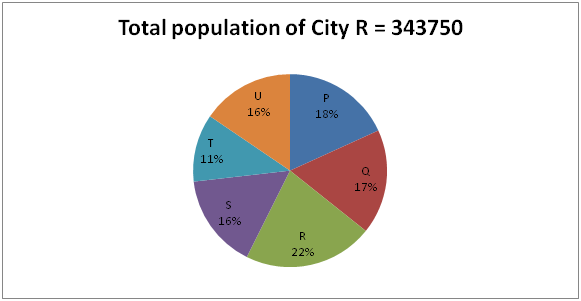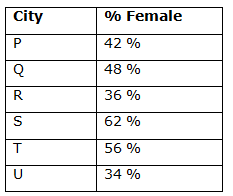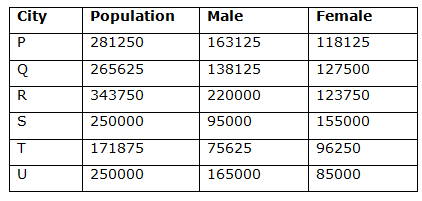# IBPS PO Mains 2018 – Quantitative Aptitude Questions Day-29

Dear Readers, Bank Exam Race for the Year 2018 is already started, To enrich your preparation here we have providing new series of Practice Questions on Quantitative Aptitude – Section. Candidates those who are preparing for IBPS PO Mains 2018 Exams can practice these questions daily and make your preparation effective.

[WpProQuiz 4309]

1) P, Q and R started a business by investing in the ratio of 2: 5: 6. After 3 months, P invested Rs. 5000 more and after another 5 months R withdraw Rs. 10000. Find the initial investment of P at the end of the year, if the share of P, Q and R is in the ratio of 81: 180: 208?

a) Rs. 30000

b) Rs. 20000

c) Rs. 24000

d) Rs. 26000

e) None of these

2) Rajesh borrowed Rs. 80000 from a bank at a rate of 10 % per annum for two years at simple interest and lends the same money to Sree at the rate of 10 % for two years but he charged compound interest. What is the overall gain of Rajesh?

a) Rs. 650

b) Rs. 950

c) Rs. 800

d) Rs. 400

e) None of these

3) 10 men and 21 women can complete a piece of work in 12 days while 8 men and 12 women can complete the same work in 18 days. 12 men and 10 women to complete the same work in how many days?

a) 12 4/9 days

b) 15 3/7 days

c) 13 5/6 days

d) 16 2/3 days

e) None of these

4) A shopkeeper sells an item at a profit of 25%. If he reduces the selling price of the item by Rs. 1400, he incurs a loss of 10%. Find the cost price of the item?

a) Rs. 5200

b) Rs. 2800

c) Rs. 3600

d) Rs. 4000

e) None of these

5) The speed of the man in still water is 15 km/hr and the rate of flow of water is 7 km/hr. He takes 7 ½ hours to row to a place and come back. Find the distance between these two places?

a) 72 Km

b) 96 Km

c) 108 Km

d) 44 Km

e) None of these

Directions (Q. 6 – 10) Study the following information carefully and answer the given questions:

The following pie chart shows the percentage distribution of total population of six different cities in a certain state and the table shows the percentage of female in the citi6) Find the total number of males in all six cities together?

a) 735425

b) 912560

c) 793650

d) 856875

e) None of these

7) Total population of City P and Q is approximately what percentage more/less than the total population of City S and T?

a) 30 %

b) 51 %

c) 34 %

d) 42 %

e) 66 %

8) Find the ratio between the total female population of City T and U together to that of total male population of City Q and R together?

a) 193: 272

b) 290: 573

c) 253: 478

d) 178: 351

e) None of these

9) Find the difference between the total male population of all the six cities to that of total female population of all six cities?

a) 178560

b) 165420

c) 151250

d) 143280

e) None of these

10) The total male population of City R and S together is approximately what percentage of total female population of City Q and T together?

a) 125 %

b) 140 %

c) 115 %

d) 175 %

e) 90 %

The share of P, Q and R,

= > [2x*3 + (2x + 5000)*9]: [5x*12]: [6x*8 + (6x – 10000)*4]

= 81: 180 : 208

= > [6x + 18x + 45000] : [60x] : [24x – 40000] = 81 : 180 : 208

= > [24x + 45000] : [60x] : [24x – 40000] = 81 : 180 : 208

According to the question,

(24x + 45000)/60x = 81/180

24x + 45000 = 27x

3x = 45000

X = 15000

The initial investment of P = 2x = Rs. 30000

Here gain is the difference between simple interest and compound interest.

Difference = Sum*(r/100)2

= > 80000*(10/100)2

= > 80000*(1/100)

= > 800

Overall gain = Rs. 800

Here work equal. So,

(10m + 21w)*12 = (8m + 12w)*18

20m + 42 w = 24 m + 36 w

6 w = 4 m

3 w = 2 m

1 m = (3/2) w

10m + 21w = 10*(3/2)w + 21w = 15w + 21w = 36w

12m + 10w = 12*(3/2)w + 10w = 18w + 10w = 28w

Women      days

36               12

28                ?

36*12 = 28*x

X = (36*12)/28 = 15 3/7 days

Let the cost price of the item be x,

According to the question,

125 % of x – 90 % of x = 1400

35% of x = 1400

35x/100 = 1400

x = 1400*100/35

x = 4000

Cost price of the item = Rs. 4000

Let the distance be x,

Speed of man in still water = 15 km/hr

Speed of stream = 7 km/hr

Speed of upstream = 15 – 7 = 8 km/hr

Speed of downstream = 15 + 7 = 22 km/hr

According to the question,

(x/22) + (x/8) = 7 ½

30x/(22*8) = 15/2

X = 44 Km

Directions (6-10):

Total population of City R = 343750

22 % of total Population of state = 343750

(22/100)*x = 343750

X = 343750*(100/22) = 1562500Total male population of all six cities,

= > 163125 + 138125 + 220000 + 95000 + 75625 + 165000

= > 856875

Required % = {[(18 + 17) – (16 + 11)]/(16 + 11)}*100

= [(35- 27)/27]*100

= > (8/27)*100 = 29.63 % = 30 %

The total female population of City T and U

= > 96250 + 85000 = 181250

The total male population of City Q and R

= > 138125 + 220000 = 358125

Required ratio = 181250: 358125 = 290: 573

Total male population of all six cities,

= > 163125 + 138125 + 220000 + 95000 + 75625 + 165000

= > 856875

Total female population of all six cities

= > 1562500 – 856875 = 705625

Required difference = 856875 – 705625 = 151250

The total male population of City R and S

= > 220000 + 95000 = 315000

Total female population of City Q and T

= > 127500 + 96250 = 223750

Required % = (315000/223750)*100 = 140 %

Daily Practice Test Schedule | Good Luck

 Topic Daily Publishing Time Daily News Papers & Editorials 8.00 AM Current Affairs Quiz 9.00 AM Current Affairs Quiz (Hindi) 9.30 AM IBPS Clerk Prelims – Reasoning 10.00 AM IBPS Clerk Prelims – Reasoning (Hindi) 10.30 AM IBPS Clerk Prelims – Quantitative Aptitude 11.00 AM IBPS Clerk Prelims – Quantitative Aptitude (Hindi) 11.30 AM Vocabulary (Based on The Hindu) 12.00 PM IBPS Clerk Prelims – English Language 1.00 PM SSC Practice Questions (Reasoning/Quantitative aptitude) 2.00 PM IBPS PO/Clerk – GK Questions 3.00 PM SSC Practice Questions (English/General Knowledge) 4.00 PM Daily Current Affairs Updates 5.00 PM IBPS PO Mains – Reasoning 6.00 PM IBPS PO Mains – Quantitative Aptitude 7.00 PM IBPS PO Mains – English Language 8.00 PM